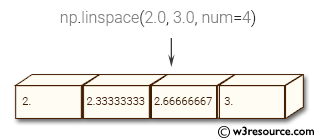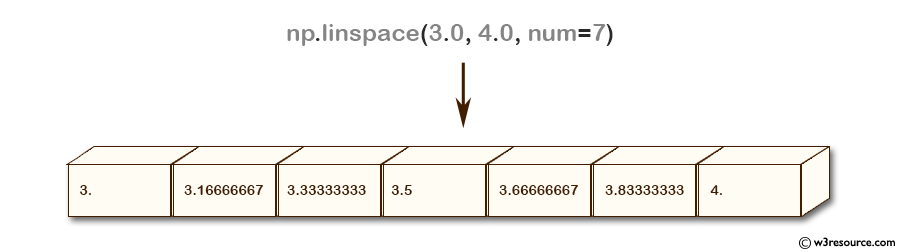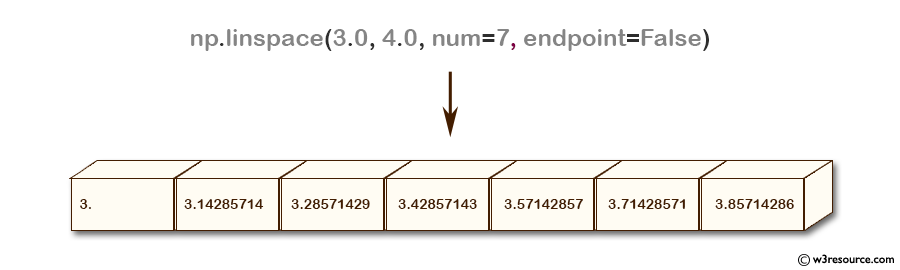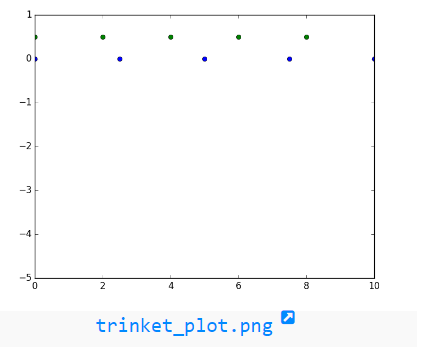# NumPy: numpy.linspace() function

## numpy.linspace() function

The numpy.linspace() function is used to create an array of evenly spaced numbers within a specified range. The range is defined by the start and end points of the sequence, and the number of evenly spaced points to be generated between them.

Syntax:

`numpy.linspace(start, stop, num=50, endpoint=True, retstep=False, dtype=None)`Parameters:

Name Description Required /
Optional
start The starting value of the sequence. Required
stop The end value of the sequence, unless endpoint is set to False. In that case, the sequence consists of all but the last of num + 1 evenly spaced samples, so that stop is excluded. Note that the step size changes when endpoint is False. Required
num Number of samples to generate. Default is 50. Must be non-negative. Optional
endpoint If True, stop is the last sample. Otherwise, it is not included. Default is True. Optional
retstep If True, return (samples, step), where step is the spacing between samples. Optional
dtype The type of the output array. If dtype is not given, infer the data type from the other input arguments.
New in version 1.9.0.
Optional

Return value:

ndarray - There are num equally spaced samples in the closed interval [start, stop] or the half-open interval [start, stop) (depending on whether endpoint is True or False).

step : float, optional - Only returned if retstep is True
Size of spacing between samples.

Example: Generating evenly spaced values with numpy linspace()

``````>>> import numpy as np
>>> np.linspace(3.0, 4.0, num=7)
array([ 3.        ,  3.16666667,  3.33333333,  3.5       ,  3.66666667,
3.83333333,  4.        ])
>>> np.linspace(3.0,4.0, num=7, endpoint=False)
array([ 3.        ,  3.14285714,  3.28571429,  3.42857143,  3.57142857,
3.71428571,  3.85714286])
>>> np.linspace(3.0,4.0, num=7, retstep=True)
(array([ 3.        ,  3.16666667,  3.33333333,  3.5       ,  3.66666667,
3.83333333,  4.        ]), 0.16666666666666666)
``````

The above code demonstrates the usage of numpy's linspace() function for generating evenly spaced values within a specified interval. The function takes three main arguments: start point, end point, and the number of values to be generated.
In the first example, linspace() generates 7 values between 3.0 and 4.0, including the endpoint of 4.0.
In the second example, the endpoint is excluded by setting the optional parameter 'endpoint' to False, and linspace() generates 7 values with equal spacing between 3.0 and 4.0.
In the third example, the optional parameter 'retstep' is set to True, which returns a tuple containing the generated array and the step size between adjacent values.

Pictorial Presentation:Example: Plotting Points with Matplotlib

``````>>> import numpy as np
>>> import matplotlib.pyplot as plt
>>> A = 5
>>> x = np.zeros(A)
>>> a1 = np.linspace(0, 10, A, endpoint=True)
>>> a2 = np.linspace(0, 10, A, endpoint=False)
>>> plt.plot(a1, x, 'o')
[<matplotlib.lines.Line2D object at 0x7f3d13a48080>]
>>> plt.plot(a2, x + 0.5, 'o')
[<matplotlib.lines.Line2D object at 0x7f3d1b582438>]
>>> plt.ylim([-5.0, 1])
(-5.0, 1)
>>> plt.show()
``````

The above code uses the numpy and matplotlib.pyplot libraries to create a simple plot of points.
1. A is set to 5 and x is created as a numpy array of 5 zeros.
2. Two arrays a1 and a2 are created using numpy.linspace method, which returns evenly spaced numbers over a specified interval.
3. plt.plot is used to create two sets of points on the plot.

numpy.linspace.plot showPython - NumPy Code Editor:

Previous: arange()
Next: logspace()

﻿